# sparrpowR: Power analysis to detect spatial relative risk clusters

#### 6/10/2020

Start with the necessary packages and seed for the vignette

``````loadedPackages <- c("broom", "geojsonio", "ggmap", "ggplot2", "grDevices", "maptools", "raster", "rgdal", "rgeos", "sp", "sparrpowR", "spatstat", "tibble")
invisible(lapply(loadedPackages, require, character.only = TRUE))
set.seed(1234) # for reproducibility``````

Import data from Open Data DC website

``````# Washington, D.C. boundary
dc <- geojsonio::geojson_read(gis_path1,  what = "sp")

# American Community Survey 2018 Census Tracts
gis_path2 <- "https://opendata.arcgis.com/datasets/faea4d66e7134e57bf8566197f25b3a8_0.geojson"
census <- geojsonio::geojson_read(gis_path2,  what = "sp")``````

We want to create a realistic boundary (i.e., polygon) of our study area. We are going to spatially clip our DC boundary by the census tracts in an attempt to remove major bodies of water where people do not reside.

``````clipwin <- maptools::unionSpatialPolygons(census, IDs = rep(1, length(census)))
dcc <- rgeos::gIntersection(dc, clipwin, byid = TRUE)
# Plot
plot(dc, main = "DC Boundary")
plot(census,  main = "American Community Survey\n2018")
plot(dcc, main = "Clipped Boundary")``````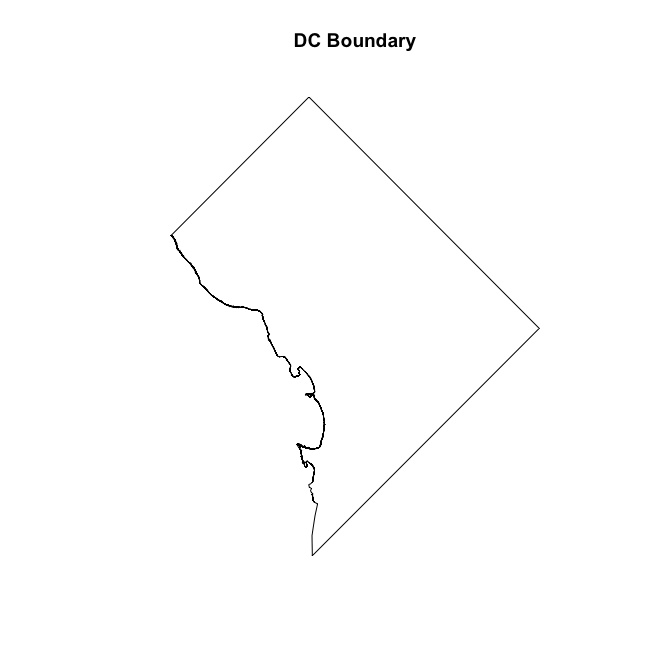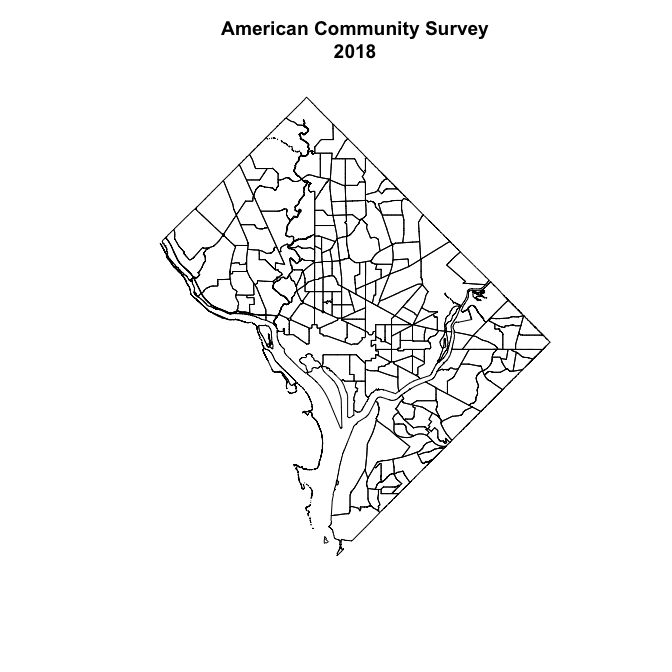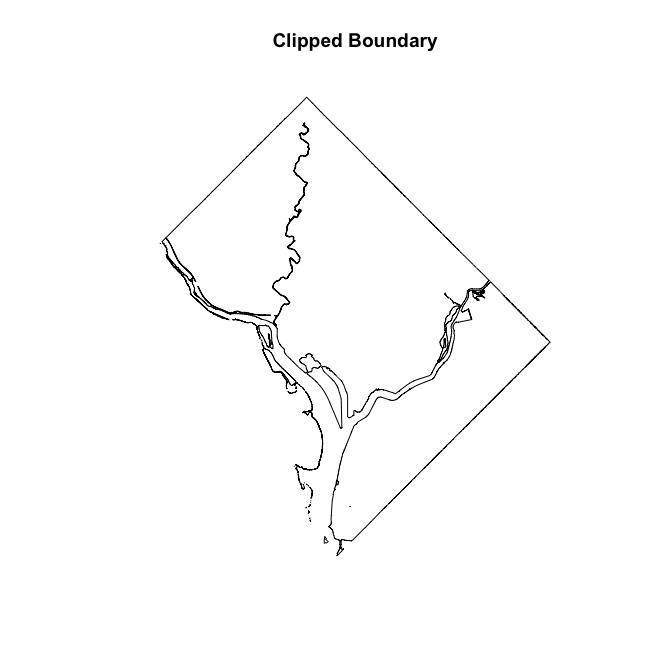Our developed method, `sparrpowR`, relies on the `spatstat` package to simulate data, which assumes point locations are on a planar (i.e., flat) surface. Our boundary is made up of geographical coordinates on Earth (i.e., a sphere), so we need to flatten our boundary by spatially projecting it with an appropriate spatial reference system (CRS). For the District of Columbia, we are going to use the World Geodetic System 1984 (WGS84) Universal Transverse Mercator (UTM) Zone 18N EPSG:32619. We then convert the boundary into a `owin` object required by the `spatstat` package

``````dcp <- sp::spTransform(dcc, CRSobj = sp::CRS(projargs = "+init=EPSG:32618"))
dco <- spatstat::as.owin(dcp)``````

In this hypothetical example, we want to estimate the local power of detecting a spatial case cluster relative to control locations. Study participants that tested positive for a disease (i.e., cases) are hypothesized to be located in a circular area around the Navy Yard, an Environmental Protection Agency (EPA) Superfund Site (see the success story).

``````navy <- data.frame(lon = 326414.70444451, lat = 4304571.1539442)
spf <- sp::SpatialPoints(coords = navy, proj4string = sp::CRS(projargs = "+init=EPSG:32618"))
# Plot
sp::plot(dcp, main = "Location of Hypothetical\nDisease Cluster")
sp::plot(spf, col = "red", add = T)
legend("bottom", xpd = T, y.intersp = -1.5, legend = c("Navy Yard"), col = "red", pch = 3, bty = "n")``````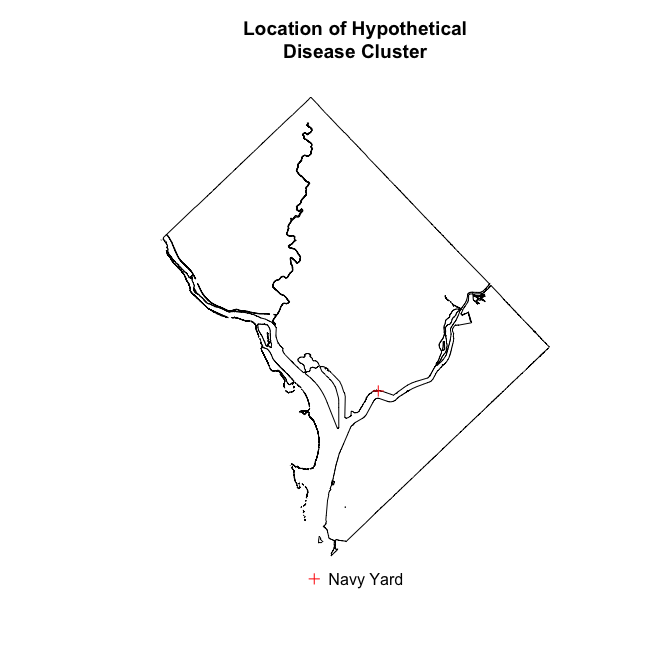We will assume that approximately 50 cases (e.g., `n_case = 50`) were clustered around the center of the Navy Yard (e.g., `samp_case = "MVN"`) with more cases near the center and fewer cases about 1 kilometers away (e.g., `s_case = 1000`).

If we were to conduct a study, where would we be sufficiently statistically powered to detect this spatial case cluster? To answer this question we will randomly sample approximately 950 participants (e.g., `n_conrol = 950` or 5% disease prevalence) around the Navy Yard (e.g., `samp_control = "MVN"`) sampling more participants near the center and fewer participants about 2 kilometers away (e.g., `s_control = 2000`). These participants would test negative for a disease (i.e., controls) were we to conduct a study. We can then resample control locations iteratively, as if we conducted the same study multiple times (e.g., `sim_total = 10`). We can conclude that we are sufficiently powered to detect a spatial case cluster in areas where a statistically significant (e.g., two-tailed alpha level of 0.05 or `lower_tail = 0.025`) spatial case cluster was located in at least 80% (e.g., `p_thresh = 0.8`) of these theoretical studies.

``````start_time <- Sys.time() # record start time
sim_power <- spatial_power(x_case = navy[], y_case = navy[], # center of cluster
x_control = navy[], y_control = navy[], # center of cluster
n_case = 50, n_control = 950, # sample size of case/control
samp_case = "MVN", samp_control = "MVN", # samplers
s_case = 1000, s_control = 2000, # approximate size of clusters
cascon = FALSE, # power for case cluster(s) only
lower_tail = 0.025, upper_tail = 0.975, # two-tailed alpha
sim_total = 1, # number of iterations
win = dco, # study area
resolution = 100, # number gridded knots on x-axis
edge = "diggle", # correct for edge effects
adapt = FALSE, # fixed-bandwidth
h0 = NULL, # automatically select bandwidth for each iteration
verbose = FALSE) # no printout
end_time <- Sys.time() # record end time
time_srr <- end_time - start_time # Calculate run time``````

The process above took about 9.9 minutes to run. Of the 1 iterations, we simulated 40 case locations and an average 774 (SD: NA) control locations for an average prevalence of 5.17% (SD: NA%). The averaged bandwidth for the statistic was 0.8 kilometers (SD: NA).

``````cols <- c("cornflowerblue", "green", "red", "lightgreen", "blue") # colors for plots
chars <- c(4,5) # symbols for point-locations
sizes <- c(0.5,0.5) # size of point-locations
p_thresh <- 0.8 # 80% of iterations with statistically significant results

## Data Visualizaiton of Input and Power
spatial_plots(input = sim_power, # use output of above simulation
p_thresh = p_thresh, # power cut-off
plot_pts = TRUE, # display the points in the second plot
chars = chars, # case, control
sizes = sizes, # case, control
cols = cols) # colors of plot``````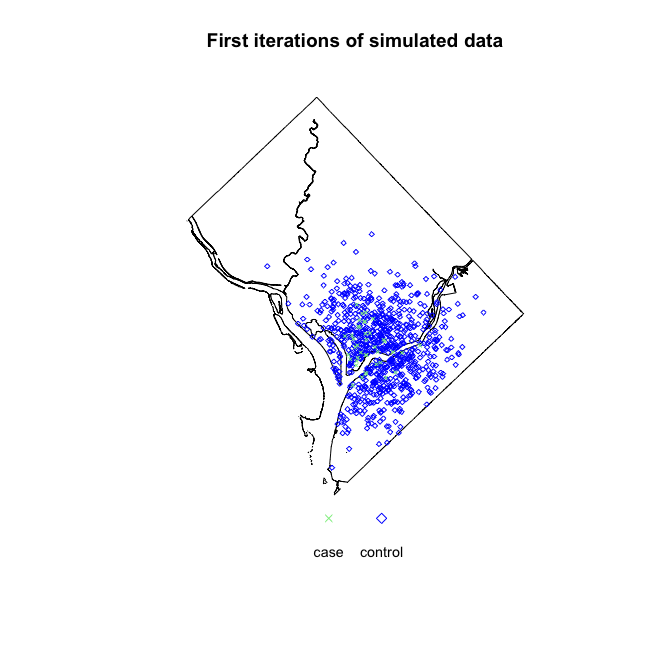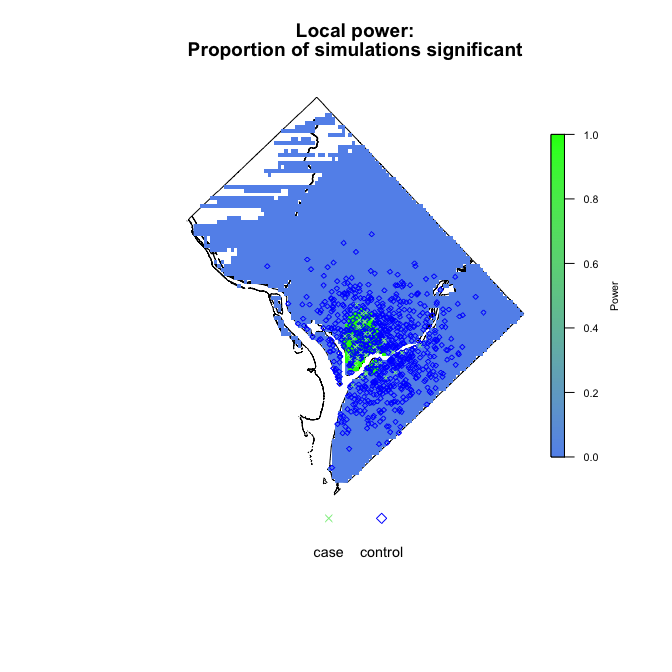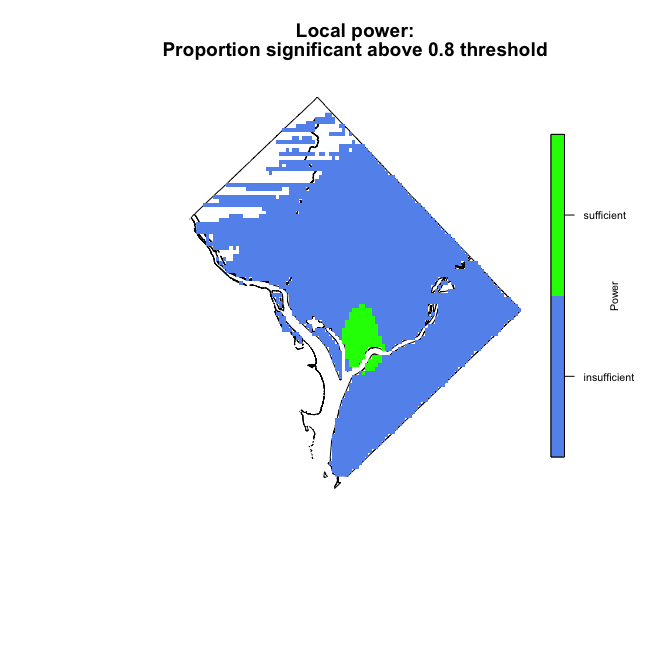Now, lets overlay our results on top of a basemap. Here, we will use an open-source map from Stamen, that is unprojected in WGS84. We extract the rectangular box (i.e., bounding box) surrounding our polygon boundary of the District of Columbia (WGS84) and enlarge it using the custom function `expandbb` to the size of basemap we want to import.

``````expandbb <- function(bb, f) {
x <- bb - bb # range of x values
y <- bb - bb # range of y values
nb <- bb # make a copy
nb <- bb - (f * x) # xmin - left
nb <- bb + (f * x) # xmax - right
nb <- bb - (f * y) # ymin - bottom
nb <- bb + (f * y) # ymax - top
return(nb)}
dcbb <- expandbb(bb = sp::bbox(dc), f = 0.01)
base_map <- ggmap::get_map(location = dcbb, maptype = "toner", source = "stamen")``````

Prepare the points from the first simulation for plotting in `ggplot2` suite.

``````sim_pts <- sim_power\$sim  # extract points from first iteration
sim_pts <- maptools::as.SpatialPointsDataFrame.ppp(sim_pts) # convert to spatial data frame
raster::crs(sim_pts) <- sp::proj4string(dcp) # set initial projection
sim_pts_wgs84 <-  sp::spTransform(sim_pts, CRSobj = sp::CRS(projargs = "+init=epsg:4326")) # project to basemap
sim_pts_df <- tibble::tibble(data.frame(sim_pts_wgs84)) # convert to tidy data frame``````

Prepare the original boundary for plotting in `ggplot2` suite.

``````dc_df <- broom::tidy(dcc) # conver to a tidy dataframe
dcc\$polyID <- sapply(slot(dcc, "polygons"), function(x) slot(x, "ID")) # preserve polygon id for merge
dc_df <- merge(dc_df, dcc, by.x = "id", by.y="polyID") # merge data``````

Prepare the raster from the simulation for plotting in `ggplot2` suite.

``````pvalprop <- tibble::tibble(x = sim_power\$rx, y = sim_power\$ry,
z = sim_power\$pval_prop) # extract proportion significant
lrr_narm <- na.omit(pvalprop) # remove NAs
sp::coordinates(lrr_narm) <- ~ x + y # coordinates
gridded(lrr_narm) <- TRUE # gridded
pvalprop_raster <- raster::raster(lrr_narm) # convert to raster
rm(pvalprop, lrr_narm) # conserve memory
raster::crs(pvalprop_raster) <- raster::crs(dcp) # set output project (UTM 18N)
pvalprop_raster <- raster::projectRaster(pvalprop_raster, crs = raster::crs(dc)) # unproject (WGS84)
rtp <- raster::rasterToPolygons(pvalprop_raster) # convert to polygons
rtp@data\$id <- 1:nrow(rtp@data)   # add id column for join
rtpFort <- broom::tidy(rtp, data = rtp@data) # convert to tibble
rtpFortMer <- merge(rtpFort, rtp@data, by.x = 'id', by.y = 'id')  # join data
rampcols <- grDevices::colorRampPalette(colors = c(cols, cols), space="Lab")(length(raster::values(pvalprop_raster))) # set colorramp``````

Plot local power as a continuous outcome with point-locations using the `ggplot2` suite.

``````ggmap::ggmap(base_map) + # basemap
ggplot2::geom_polygon(data = dc_df, # original boundary
ggplot2::aes(x = long, y = lat, group = group),
fill = "transparent",
colour = "black") +
ggplot2::geom_polygon(data = rtpFortMer, # output raster as polygons
ggplot2::aes(x = long, y = lat, group = group, fill = z),
size = 0,
alpha = 0.5) +
ggplot2::scale_fill_gradientn(colours = rampcols) + # colors for polygons
ggplot2::geom_point(data = sim_pts_df, # simulated point-locations
ggplot2::aes(x = mx, y = my, color = marks, shape = marks),
alpha = 0.8) +
ggplot2::scale_color_manual(values = cols[4:5]) + # fill of point-locations
ggplot2::scale_shape_manual(values = chars) + # shope of point-locations
ggplot2::labs(x = "", y = "", fill = "Power", color = "", shape = "") # legend labels``````